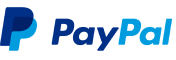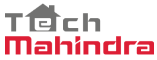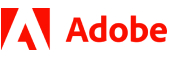New update is available. Click here to update.
Topics

# Count Even Odd

Easy0/40
Average time to solve is 10m+2 more companies

## Problem statement

You have been given an array/list of integers 'ARR' of size 'N'. Your task is to find two things-

1. The number of elements that are occurring an Odd number of times.

2. The number of elements that are occurring Even a number of times.

For Example:
``````ARR = [2, 1, 2, 1, 5, 5, 2]
Output: 1 2

2 occurs three(odd) times.
1 occurs two(even) times.
5 occurs two(even) times.

So, the total 1 element is occurring an odd number of times and 2 elements are occurring an even number of times.
``````
Detailed explanation ( Input/output format, Notes, Images )
Constraints:
``````1 <= T <= 10^2
0 <= N <= 5 * 10^3
1 <= ARR[i] <= 10^9

Time Limit: 1 sec
``````
Output Format:
``````For each test case, print two single space-separated integers representing the number of odd occurring elements and number of even occurring elements respectively.

The output of each test case will be printed a separate line.
``````

Note:

``````You are not required to print the output, it has already been taken care of. Just implement the function.
``````
Sample Input 1:
``````2
5
4 5 1 2 1
4
2 1 2 1
``````
Sample Output 1:
``````3 1
0 2
``````
Explanation for Sample 1:
``````In the first test case, three integers(4, 5 and 2) occur odd times and the only integer 1 occurs even times.

In the second test case, no integer occurs odd times and two integers(1 and 2) occurs even times.
``````
Sample Input 2:
``````1
8
5 2 9 9 7 5 1 3
``````
Sample Output 2:
``````4 2
``````Console